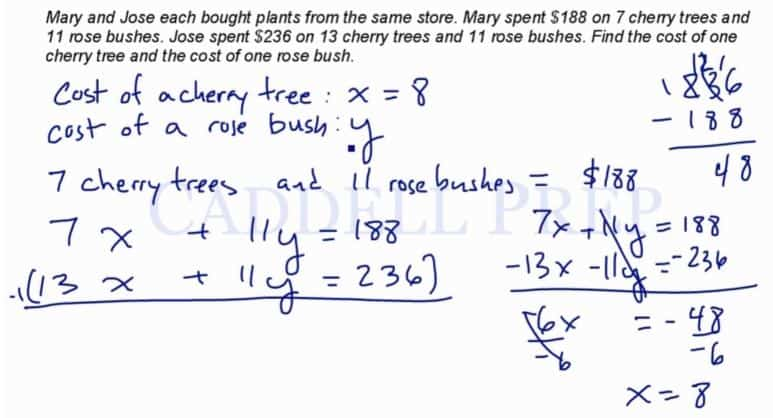# Word Problems – System Of Equations

To solve a word problem using a system of equations, it is important to;
– Identify what we don’t know
– Declare variables
– Use sentences to create equations

An example on how to do this:

Mary and Jose each bought plants from the same store. Mary spent \$188 on 7 cherry trees and 11 rose bushes. Jose spent \$236 on 13 cherry trees and 11 rose bushes. Find the cost of one cherry tree and the cost of one rose bush.

Cost of a cherry tree:$x$
Cost of a rose bush:$y$
7 cherry trees and 11 rose bushes = \$188$7x + 11y = 188$$13x + 11y = 236$$7x + 11y = 188$$\underline {-13x - 11y = -236 }$

The y-values cancel each other out, so now you are left with only x-values and real numbers.$-6x = -48$$x = 8$

Then, you plug in your x-value into an original equation in order to find the y-value.$7x + 11y = 188$$7(8) + 11y = 188$$56 + 11y = 188$$11y = 132$$y = 12$

Cost of a cherry tree: \$8
Cost of a rose bush: \$12

## Video-Lesson Transcript

To solve a word problem using system of equations, it is important to:
1. Identify what we don’t know
2. Declare variables.
3. Use sentences to create equations.

Let’s have an example:

Mary and Jose each bought plants from the same store. Mary spent$\188$ on$7$ cherry trees and$11$ rose bushes. Jose spent$\236$ on$13$ cherry trees and$11$ rose bushes. Find the cost of one cherry tree and the cost of one rose bush.

Let’s solve this by following steps above.

1. What we don’t know:
cost of a cherry tree
cost of a rose bush

2. Declare variables:
cost of a cherry tree :$x$
cost of a rose bush :$y$

3. Use sentences to create equations.

For Mary:$7$ cherry trees and$11$ rose bushes$= \188$$7x + 11 y = 188$

For Jose:$13$ cherry trees and$11$ rose bushes$= \236$$13x + 11 y = 236$

Now, we have a system of equations$7x + 11y = 188$$13x + 11y = 236$

We can solve this by process of substitution, elimination or fraction.

Since the value of$y$ is the same for both equations, let’s do the process of elimination.

First, let’s multiply the first equation by$-1$$-1 (13x + 11y = 236)$

Here we’ll have a negated equation$-13x - 11y = -236$

Let’s do the process of elimination now$7x + 11y = 188$$+ {-13x} + 11y = 236$

We’ll have$6x = -48$

Then, let’s isolate$x$ by dividing both sides by$-6$$\dfrac{-6x}{-6} = \dfrac{-48}{-6}$

Now, we have$x = 8$

Remember, our declared variable?

cost of a cherry tree :$x$

Since$x = 8$

Now we can say that

cost of a cherry tree :$= 8$Now, let’s solve for the value of$y$ by getting one equation and plugging the value of$x$.

Let’s use the first equation to plug in$x = 8$$7x + 11y = 188$$7(8) + 11y = 188$$56 + 11y = 188$

Let’s isolate$y$ by subtracting$56$ on both sides of the equation$56 - 56 + 11y = 188 - 56$$11y = 132$

Then divide by$11$$\dfrac{11y}{11} = \dfrac{132}{11}$

And we get$y = 12$

Now, we know that cost of a rose bush is$12$.